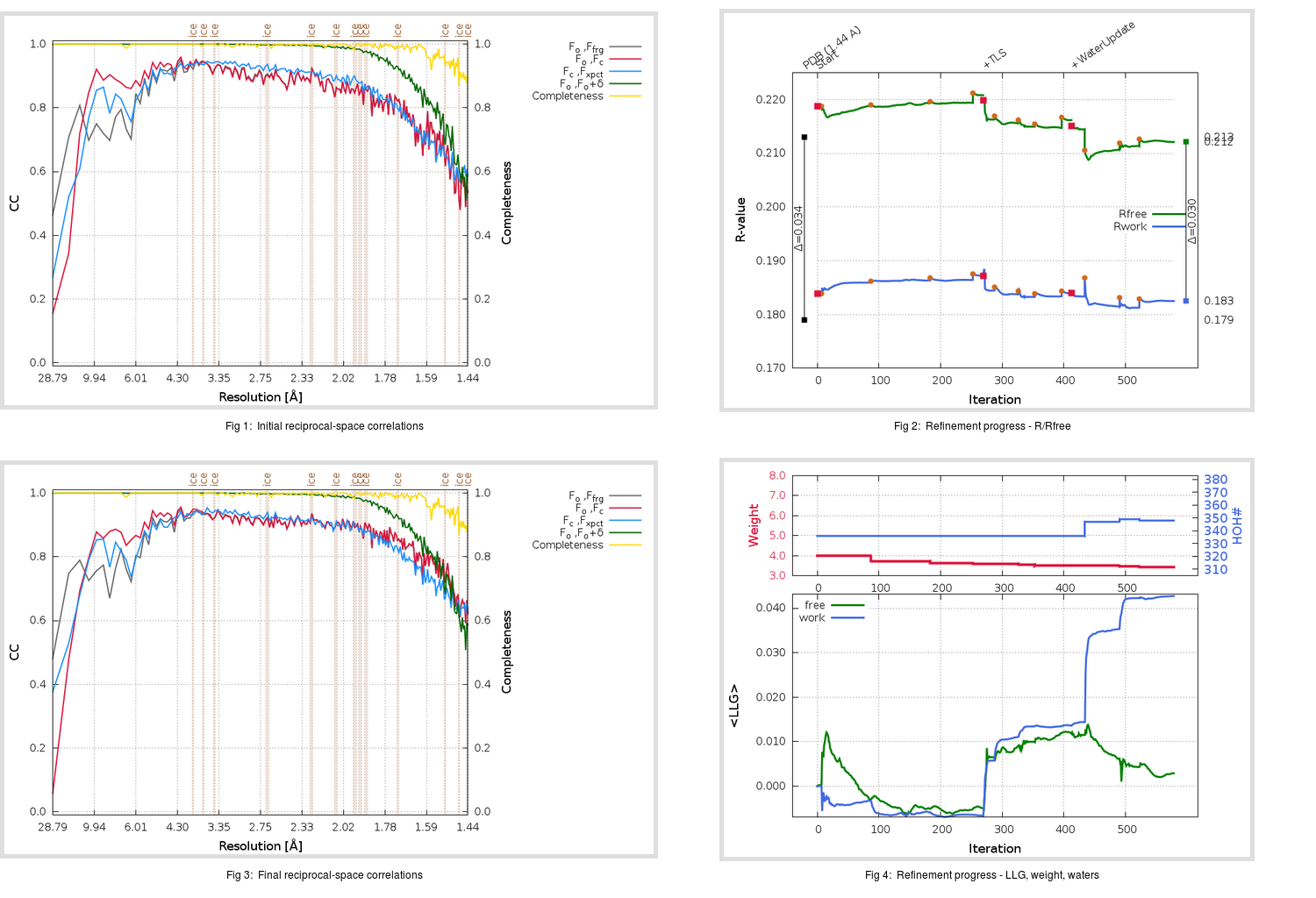Content:

```    Diffraction limits & principal axes of ellipsoid fitted to diffraction cut-off surface:
1.589         0.9571   0.0000  -0.2898       0.980 a* - 0.201 c*
1.385         0.0000   1.0000   0.0000       b*
1.388         0.2898   0.0000   0.9571       0.647 a* + 0.762 c*
```

## Deposited

` `
 Date deposited Date data collection Resolution R, Rfree 20200315 20200305 1.44 0.1770 0.2130

Molprobity (CCP4 7.0 version) summary:

```Ramachandran outliers =   0.33 %
favored =  97.68 %
Rotamer outliers      =   0.38 %
C-beta deviations     =     0
Clashscore            =   3.37
RMS(bonds)            =   0.0140
RMS(angles)           =   1.85
MolProbity score      =   1.20
Resolution            =   1.44
R-work                =   0.1770
R-free                =   0.2130
```

```Number of waters      =   336

<B> (all atoms) =   20.46 ( sd =    9.10 ) for       2735 non-hydrogen atoms
<B>   (protein) =   18.67 ( sd =    7.35 ) for       2370 non-hydrogen atoms
<B>     (water) =   32.25 ( sd =   10.98 ) for        336 non-hydrogen atoms
<B>    (others) =   30.24 ( sd =    8.18 ) for         29 non-hydrogen atoms

B min/max       (all non-hydrogen atoms) =    9.40 /  125.05
B min/max   (protein non-hydrogen atoms) =    9.40 /   59.97
B min/max     (water non-hydrogen atoms) =   11.15 /  125.05
B min/max     (other non-hydrogen atoms) =   20.57 /   42.67
```

## BUSTER (re-)refinement

` `

Molprobity (CCP4 7.0 version) summary:

```Ramachandran outliers =   0.33 %
favored =  99.01 %
Rotamer outliers      =   1.52 %
C-beta deviations     =     0
Clashscore            =   2.11
RMS(bonds)            =   0.0115
RMS(angles)           =   1.53
MolProbity score      =   1.12
Resolution            =   1.44
R-work                =   0.1825
R-free                =   0.2121
```

```Number of waters      =   348

<B> (all atoms) =   22.21 ( sd =    8.56 ) for       2747 non-hydrogen atoms
<B>   (protein) =   20.27 ( sd =    6.53 ) for       2370 non-hydrogen atoms
<B>     (water) =   34.32 ( sd =    9.70 ) for        348 non-hydrogen atoms
<B>    (others) =   35.83 ( sd =   14.33 ) for         29 non-hydrogen atoms

B min/max       (all non-hydrogen atoms) =    8.49 /   60.60
B min/max   (protein non-hydrogen atoms) =    8.49 /   55.80
B min/max     (water non-hydrogen atoms) =   13.09 /   59.51
B min/max     (other non-hydrogen atoms) =   22.92 /   60.60
```

Refinement progression:Results:

` `
 File Remark 5RF8_aB_refine.01_03_refine.pdb.gz exact refinement commands are in header 5RF8_aB_refine.01_03_refine.mtz.gz including original deposited data and several re-refinement map coefficients 5RF8_aB_refine.01_03_BUSTER_model.cif.gz including any non-standard compound restraints 5RF8_aB_refine.01_03_BUSTER_refln.cif.gz